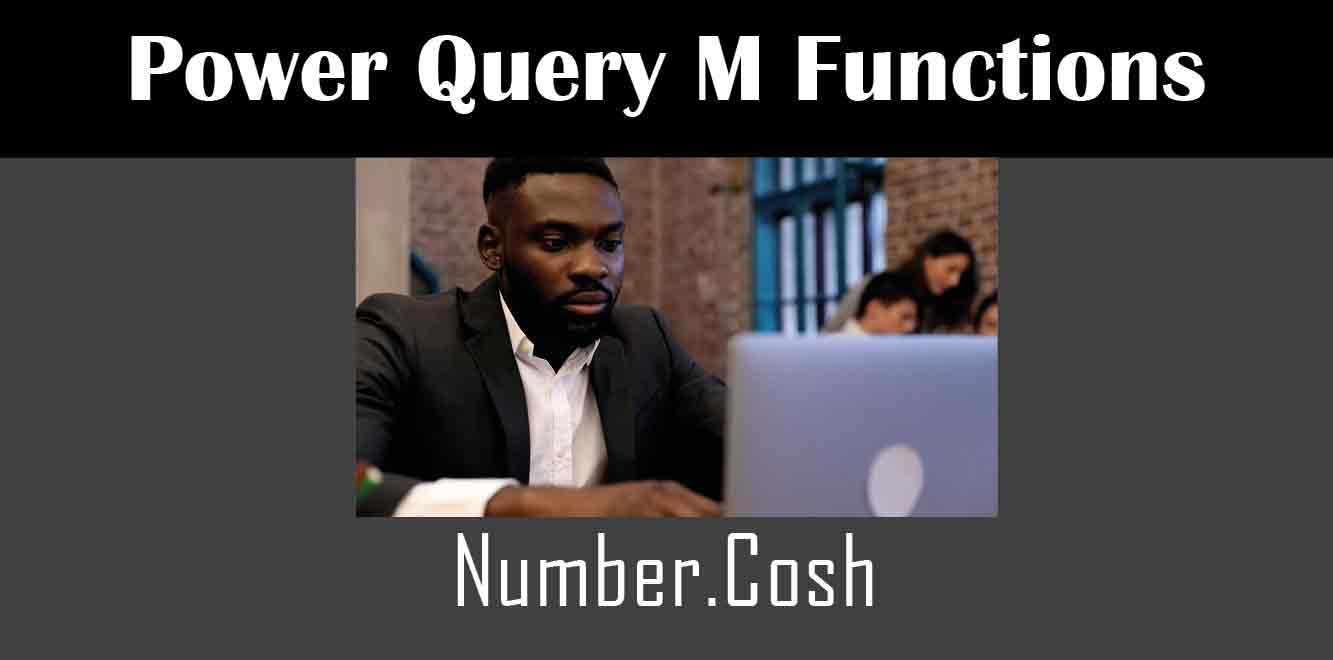# Number.Cosh

## ### What is the Number.Cosh Function?

The Number.Cosh function is used to calculate the hyperbolic cosine of a given number. The hyperbolic cosine is a mathematical function that is similar to the cosine function, but is used for hyperbolic functions. The Number.Cosh function takes a single argument, which is the number for which you want to calculate the hyperbolic cosine.

## Understanding the M Code Behind the Number.Cosh Function

To understand the M code behind the Number.Cosh function, we must first understand the M language. M is the programming language used in Power Query to manipulate data and create queries. The M code behind the Number.Cosh function is as follows:

Number.Cosh = (x) =>

let

e = exp(x),

en = exp(-x)

in

(e + en) / 2

``` Let's break down the code to better understand what is happening. The first line defines the function with a single argument, which is denoted as "x". The "=>" symbol is used to indicate that the code following it is the function's body. The next few lines are used to calculate the hyperbolic cosine. The "exp" function is used to calculate the value of "e", which is equal to the exponential of "x". The "exp" function is also used to calculate the value of "en", which is equal to the exponential of negative "x". The final line is used to calculate the hyperbolic cosine of "x". The hyperbolic cosine is calculated by adding "e" and "en" together, and then dividing the result by 2. Using the Number.Cosh Function in Power Query Now that we understand the M code behind the Number.Cosh function, let's take a look at how it can be used in Power Query. The Number.Cosh function can be used in a formula to calculate the hyperbolic cosine of a given number. For example, to calculate the hyperbolic cosine of the number 2, you would use the following formula: ```

Number.Cosh(2)

``` This formula would return the value 3.762195691. In this article, we have explored the M code behind the Number.Cosh function in Power Query. We have learned that the Number.Cosh function is used to calculate the hyperbolic cosine of a given number, and that the M code behind it uses the "exp" function to calculate the exponential of a number. We have also seen how the Number.Cosh function can be used in a formula to calculate the hyperbolic cosine of a number. The Number.Cosh function is just one of many functions available in Power Query, and understanding its M code can help you to better understand how Power Query works. Power Query and M Training Courses by G Com Solutions (0800 998 9248)Power Query and M Intensive Training Course £1,050.00 – £26,550.00 Select optionsContinue Loading DonePower Query and M Introduction £395.00 – £9,750.00 Select optionsContinue Loading DonePower Query and M Intermediate £395.00 – £9,750.00 Select optionsContinue Loading DonePower Query and M Advanced £395.00 – £9,750.00 Select optionsContinue Loading Done Upcoming Courses 20-22 Nov 23 (London or Online) Contact Us Subject Your Name (required) Company/Organisation Email (required) Telephone Training Course(s) Power BI Intensive TrainingPower BI introduction Power BI IntermediatePower BI AdvancedDAXPower Query MPower BI CertificationPower BI AdministrationPower PlatformPower AutomatePower AppsOTHER Your Message Upload Example Document(s) (Zip multiple files) ```
``` ```
``` ```
``` ```
``` ```
``` ```